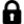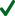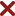£ 0 (0)LOCKED

# The North London Independent Girl's School's Consortium Group 2 11+ Practice Paper 2012 - Solved paper

This paper covers Addition and multiplication, Addition and subtraction, Coordinate plane, Data and graphs, Decimals, Division, Fractions and mixed numbers, Geometric measurement, Mixed operation, Mixed operations, Money, multiplication, Multiplication and division, Number sequences, Place values and number sense, Probability and statistics, Problem solving, Symmetry and transformations, Three dimensional figures, Time, Units of Measurement, Add and subtract whole numbers, Graph points on a coordinate plane, Pie charts, Compare decimal numbers, Put decimal numbers in order, Divide by one digit numbers, Fractions of a number, Area of compound figures, Area and Perimeter, Area and perimeter of figures on grids, Add and multiply by a onedigit number word problems, Add subtract multiply and divide, addition and multiplcation, Add subtract multiply and divide word problems, Add subtract multiply and divide whole numbers, Evaluate numerical expressions, Add and subtract money amounts, Multiply by a one digit number, Square numbers, Add subtract multiply and divide decimals, Multipication and division word problems, Use a rule to complete a number sequence, Number sequences word problems, Convert between words and digits, Calculate mean, Understanding probability, Logical problem, Guess and check problems, Multi step word problems, Multistep word problem, Multistep word problems, Logical problems, Word problems with extra or missing information, Reflect and was published by The North London Independent Girls Consortium.
There are a total of 80 questions to be attempted for 86 marks.
•
•
•
•
•
•
•
•
•
•
•
•
•
•
•
•
•
•
•
•
•
•
•
•
•
•
•
•
•
•
•
•
•
•
•
•
•
•
•
•
•
•
•
•
•
•
•
•
•
•
Answer _

Explanation

##COPY PROTECTION

All content on this page is the property of KidSmart. Please do not attempt to copy the content without prior consent from KidSmart. Any attempt to copy may lead to immediate closure of your account and no refunds would be processed.

## Best Price Comparison

Get the best price in the market and rest assured of the value.

#### Compare Single Paper Price

ProviderPriceFree Tutor SupportFree UpdatesDownload QuestionsTopic Videos1
KidSmart £6
Best Price
24/7
Best ValuePiAcademy n/aExam Paper Plus £17#### Compare Bundle Price

ProviderPriceNo. of papersFree Tutor SupportFree UpdatesTopic Videos1
KidSmart £62
Best Price
350+
Best Value
24/7PiAcademy £120 200Exam Paper Plus n/a n/a1 The topic videos are only included where the tutors felt necessary. If you need topic videos for any other topics then simply ask the tutor.

## Customer Reviews

What other parents are saying about our products.

Nilesh, Dad
"The unexpected outcome of using your past paper bundle is that my son got scholarship at Merchant Taylors. The variety of papers helped a great deal."